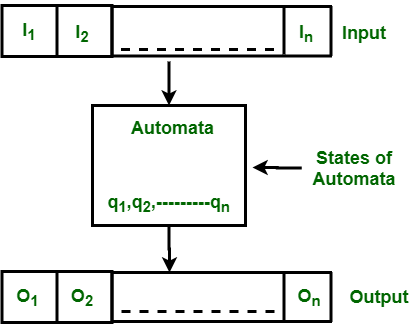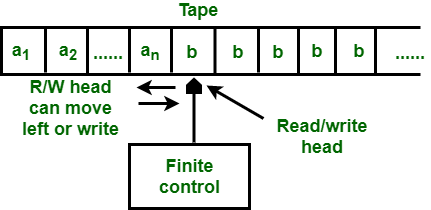Skip to content
Related Articles
Difference between Finite Automata and Turing Machine
• Last Updated : 03 Sep, 2020

1. Finite Automata :
The finite automata or finite state machine is an abstract machine which have five elements or tuple. It has a set of states and rules for moving from one state to another but it depends upon the applied input symbol. Basically it is an abstract model pf digital computer. Following figure shows some essential features of a general automation.Figure : Features of Finite Automata

The above figure shows following features of automata :

1. Input
2. Output
3. States of automata
4. State relation
5. Output relation

2. Turing Machine :
It is a powerful model which was proposed by Alan Turing in 1936. The earlier models like finite automata and push-down automata are bot considered as the accurate models because they cannot recognize simple language. But the turing machine is the most accurate model for the personal computers. A turing machine is capable of solving every problem that a real computer can do. There are also some problems which can not be solved by turing machine because these problems are beyond the theoretical limits of computation.Figure : Turing Machine Model

Difference between Finite Automata and Turing Machine :

Finite AutomataTuring Machine
It recognize the language called regular language.It will recognize not only regular language but also context free language, context sensitive language and recursively enumerable languages.
In this the input tape is of finite length from both left and right side.In this the input tape is of finite length from left but is of infinite length from right.
It consists of finite number of states, finite set of input symbols, initial state of automata and finite set of transition rules for moving from one state to another.It also contains finite set of tape symbols and a blank symbol on the tape in addition to finite number of states, finite set of input symbols, initial state of automata and finite set of transition rules for moving from one state to another.
In this head is able to move in right direction only. In two way automata, head is able to move in both directions.In this, head can move in both directions.
Head is only able to read the symbols from the tape but can not write symbols on the tape.Head is able to read as well as write symbols on the tape.
It is weak as compare to Turing Machine.It is powerful than Finite Automata.
Designing finite automata is easier.Designing turing machine is difficult and as well as complex.
The transition function in finite automata can be represented by :
δ : Q × Σ* → Q
The transition function in turing machine can be represented by :

δ : Q × T → Q × T × {L, R}

where L and R specifies the left and right movement of tape head.

Attention reader! Don’t stop learning now. Learn all GATE CS concepts with Free Live Classes on our youtube channel.

My Personal Notes arrow_drop_up